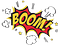# Ariadne’s thread

On 4 June 1996, only about 40 seconds after initiation of the flight sequence, at an altitude of about 3700 m, the Ariane 5 broke up and exploded.Explosion of first Ariane 5 flight, June 4, 1996 https://www.esa.int/

An independent Inquiry Board was appointed and gave out a complete report.

“ The failure of Ariane 501 was caused by the complete loss of guidance and attitude information 37 seconds after the start of the main engine ignition sequence (30 seconds after lift-off). This loss of information was due to specification and design errors in the software of the inertial reference system. The extensive reviews and tests carried out during the Ariane 5 development program did not include adequate analysis and testing of the inertial reference system or of the complete flight control system, which could have detected the potential failure.”

And in particular: “The internal SRI software exception was caused during execution of a data conversion from 64-bit floating-point to 16-bit signed integer value. The floating-point number which was converted had a value greater than what could be represented by a 16-bit signed integer. This resulted in an Operand Error. The data conversion instructions (in Ada code) were not protected from causing an Operand Error, although other conversions of comparable variables in the same place in the code were protected. “

So there was a problem converting one numeric type into another. F# solves this problem with type checking at compile time (and you can visually see it in the IDE, i.e. Visual Studio) but at the same time simplifies the life of the developer introducing “Type inference.”

Type inference refers to the automatic detection of the type of an expression in the code based on the context and previous expressions.
A clear example would be a function that sums two variables (actually in F# they are constants toward the entire life of the execution of the program):

`namespace Mahamudra.Functionsmodule Inference =let add a b = a + b + 100let a = 4let b = 6printfn "the sum %i+%i=%i" a b (add a b)let add' a b = a + b + 100.0let a' = 4.0let b' = 6.5printfn "the sum %A+%A=%A" a' b' (add' a' b')let add'' a b = List.map2 (+) a blet a'' = [1;3;4;5]let b'' = [0;1;3;6]printfn "the sum %A+%A=%A" a'' b'' (add'' a'' b'')`

The compiler infers the type of the two variables a and b and simplifies the life of the dev and at the same time enforcing the type harmonization.

The output of the previous calculations is:

`the sum 4+6=110the sum 4+6.5=110.5the sum [1; 3; 4; 5]+[0; 1; 3; 6]=[1; 4; 7; 11]`

F# goes a little further, it introduces the concept of a unit of measure.
Floating point and signed integer values can have associated units of measure. By using quantities with units, the compiler is able to verify that the arithmetic relationships have the correct units, which helps prevent programming errors.

`namespace Mahamudra.Functionsmodule UnitOfMeasure =[<Measure>] type m[<Measure>] type h[<Measure>] type sec[<Measure>] type kglet distance = 1.0<m>let time = 2.0<sec>let speed distance (time:float<sec>) = distance/time /// meter/seclet acceleration = 2.0<m/sec^2>let force = 5.0<kg m/sec^2>printfn "speed %A/%A=%A m/sec" distance time (speed distance time)`

With output: speed 1/2=0.5 m/sec.

And if we choose instead of time, the value time’ = 0.3<h>, the code won’t compile:

`/// this won't compilelet time' = 0.3<h>printfn "speed %A/%A=%A" distance time' (speed distance time')Type mismatch. Expecting a        'float<sec>'     but given a         'float<h>'     The unit of measure 'sec' does not match the unit of measure 'h'`

Now we’re sure that our code is not gonna “boom”.Pixabay License — Free for commercial use

A complete non sequitur being.

## More from Mauro Ghiani

A complete non sequitur being.